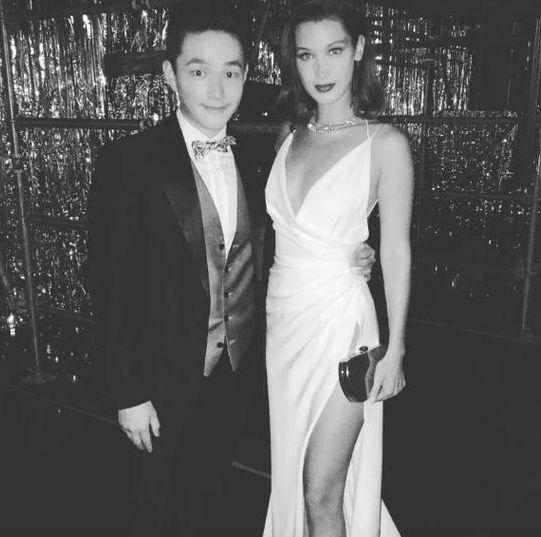1. 直接写出得数（每题1分，共15分）

15-7= 38-29= 44-33= 58+22= 36+61=

12÷2= 6÷3= 3×4= 2×5= 4×6=

1÷1= 16÷4= 0×9= 18÷6= 5÷1+9=

2. 列竖式计算（每题3分，共12分）

70-24-16= 28+36+19=

71-34+26= 64+17-25=

2.

1.4+4+4+4+4+4=4× 3+3=×2

2.被除数是16,除数是4,这个算式是。

3.写出4道乘积是12的乘法算式：、、、。

4.两个乘数都是4,积是;两个加数都是6,和是。

5.把5+5+5+5+5-4改写成乘减算式是。

6. 补充乘法口诀。

1. 有24本故事书，平均分给4个班，每个班分几本？（7分）

2. 有36元钱，每个笔记本6元钱，可以买几个笔记本？（7分）

3. 卖鸡蛋。（7分）

4. 一个书包56元，一个文具盒15元，一个魔方比一个文具盒贵5元。（8分）

（1）一个魔方多少元？

（2）小明带了100元，买完这三个物品剩下多少元？

5. 今年西西10岁，妈妈35岁。妈妈48岁时，西西多少岁？（8分）

6 2 12 10 24

1 4 0 3 14

2 . 30 83 63 56

2 . （1）3 6 （2） 6 3

2 . 16÷4

3. 3×4=12、4×3=12、2×6=12、6×2=12

4. 16 12

5. 5×4-4

6. 二（ 五 ）一十 六（ 六 ）三十六 四（ 五 ）二十

（ 三 ）四十二 （ 三 ）五十五 （ 三 ）六十八

2 . 36÷6=6（个） 答：可以买6个笔记本。

3. 70-24-31=15（个） 答：还剩15个鸡蛋。

4. （1）15+5=20（元） 答：一个魔方20元。

（2）100-20-15-56=9（元） 答：买完这三个物品剩下9元。

5. 年龄差：35-10=25（岁） 西西：48-25=23（岁）